# KSEEB Solutions for Class 7 Maths Chapter 2 Fractions and Decimals Ex 2.3

Students can Download Chapter 2 Fractions and Decimals Ex 2.3, Question and Answers, Notes Pdf, KSEEB Solutions for Class 7 Maths, Karnataka State Board Solutions help you to revise complete Syllabus and score more marks in your examinations.

## Karnataka State Syllabus Class 7 Maths Chapter 2 Fractions and Decimals Ex 2.3

Question 1.
Find :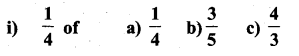Solution: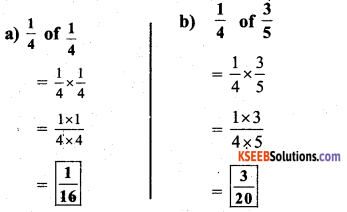ii)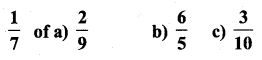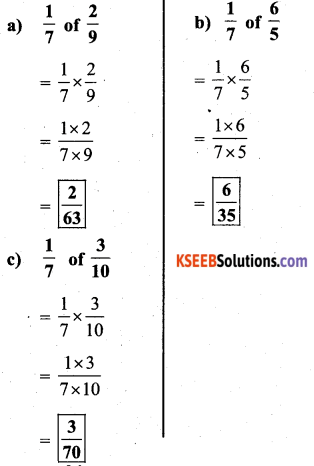Question 2.
Multiply and reduce to lowest form (if possible)
i)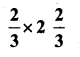Solution:ii)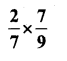Solution: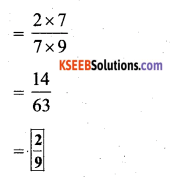iii)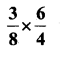Solution: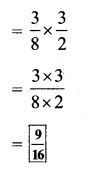iv)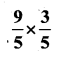Solution: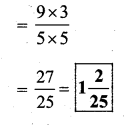v)Solution:vi)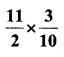Solution:vii)Solution:Question 3.
Multiply the following fractions:

i)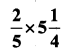Solution:ii)Solution: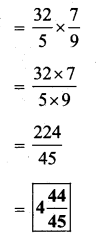iii)Solution:iv)Solution: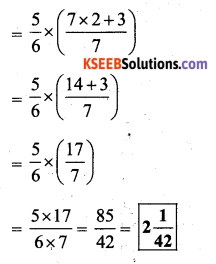v)Solution: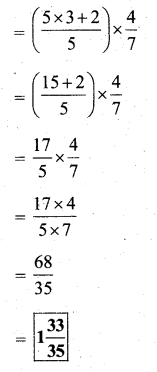vi)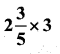Solution: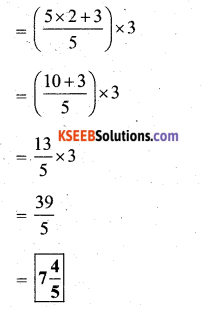vii)Solution: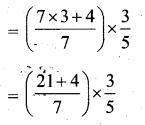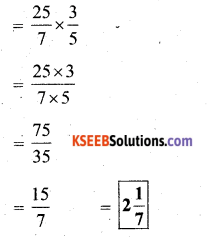Question 4.
Which is greater :
i)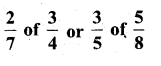Solution: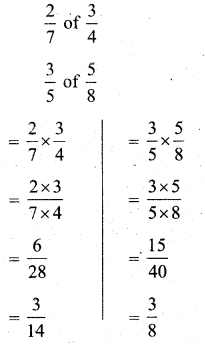Now,
we have to find greater ofTo compare fractions, we make denominator equal.ii)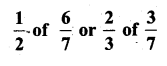Solution: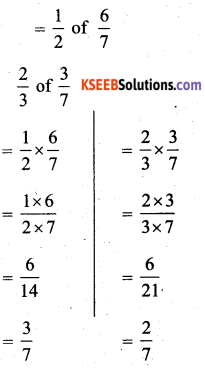Now,
we have to find greater of $$\frac{3}{7}$$ & $$\frac{2}{7}$$
Since denominator is same, number with greater numerator is greater.i.e., $$\frac{3}{7}>\frac{2}{7}$$
∴ Thus $$\frac{1}{2}$$ of $$\frac{6}{7}$$ is greaterQuestion 5.
Saili plants 4 saplings in a row, in her garden. The distance between two adjacent saplings is $$\frac{3}{4}$$m. Find the distance between the first and the last sapling.
Solution:
Saili plants 4 saplings, in a row.
The distance between each sapling = $$\frac{3}{4}$$ m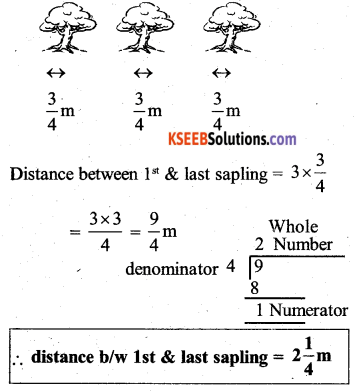Question 6.
Lipika reads a book for $$1 \frac{3}{4}$$ everyday. She reads the entire book in 6 days. How many hours in all were required by her to read the book ?
Solution:
Number of hours read everyday = $$1 \frac{3}{4}$$ hours
Number of days to read whole book = 6 days
Therefore,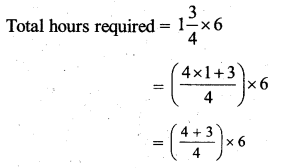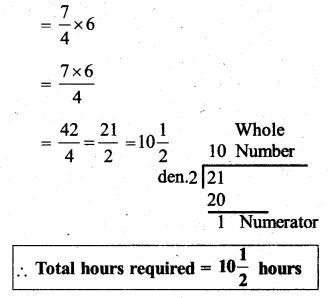Question 7.
A car runs 16 km using 1 litre of petrol. How much distance will it cover using $$2 \frac{3}{4}$$ litres of petrol.
Solution:
Distance of car runs in 1 litre = 16km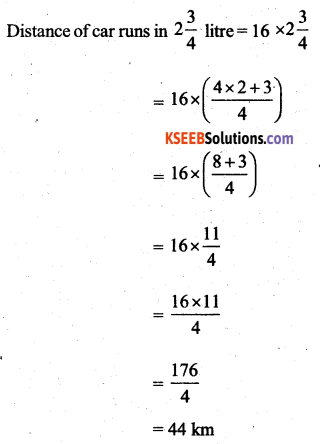∴ Car will run 44 km in $$2 \frac{3}{4}$$ litre of petrol.

Question 8.
a) i) Provide the number in the box □, such that $$\frac{2}{3} \times \square=\frac{10}{30}$$
Solution: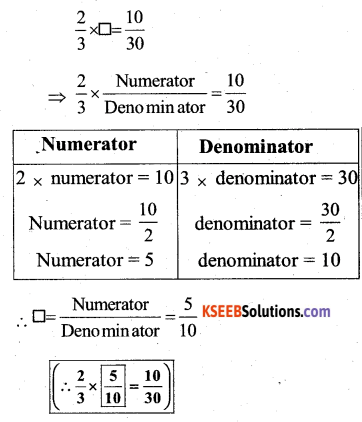ii) The simplest form of the number obtained in □ is ___
Solution:b) i) Provide the number in the box □, such that $$\frac{3}{5} \times \square=\frac{24}{75}$$
Solution: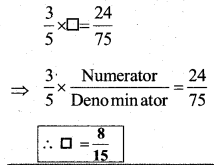b) ii) The simplest form of the number obtained in □ is ___.
Solution: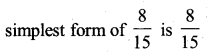it cannot be simplified further

error: Content is protected !!